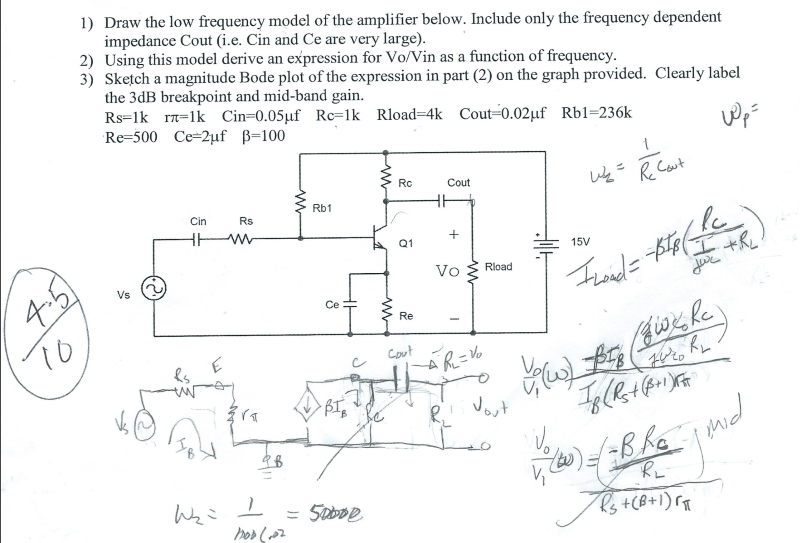Home / Answered Questions / Other / 1-draw-the-low-frequency-model-of-the-amplifier-below-include-only-the-frequency-dependent-impedance-aw734

# (Solved): 1) Draw The Low Frequency Model Of The Amplifier Below. Include Only The Frequency Dependent Impedan...1) Draw the low frequency model of the amplifier below. Include only the frequency dependent impedance Cout (i.e. Cin and Ce are very large). 2) Using this model derive an expression for Vo/Vin as a function of frequency. 3) Sketch a magnitude Bode plot of the expression in part (2) on the graph provided. Clearly label the 3dB breakpoint and mid-band gain. Rs=lk ra=lk Cin=0.05uf Rc=lk Rload=4k Cout=0.02uf Rbl=236k Re=500 Ce=2uf B=100 RC R Cout We Cout Rb1 Cin Rs + Vo 3 Road vo T Timand= pter hotel Rs +(B+1) Wza 1 I = 50000 hod (602

We have an Answer from Expert